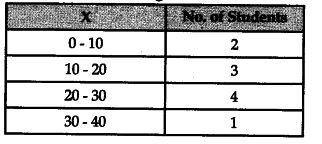# Calculate the Standard Deviation from the following data :

Calculate the Standard Deviation from the following data :